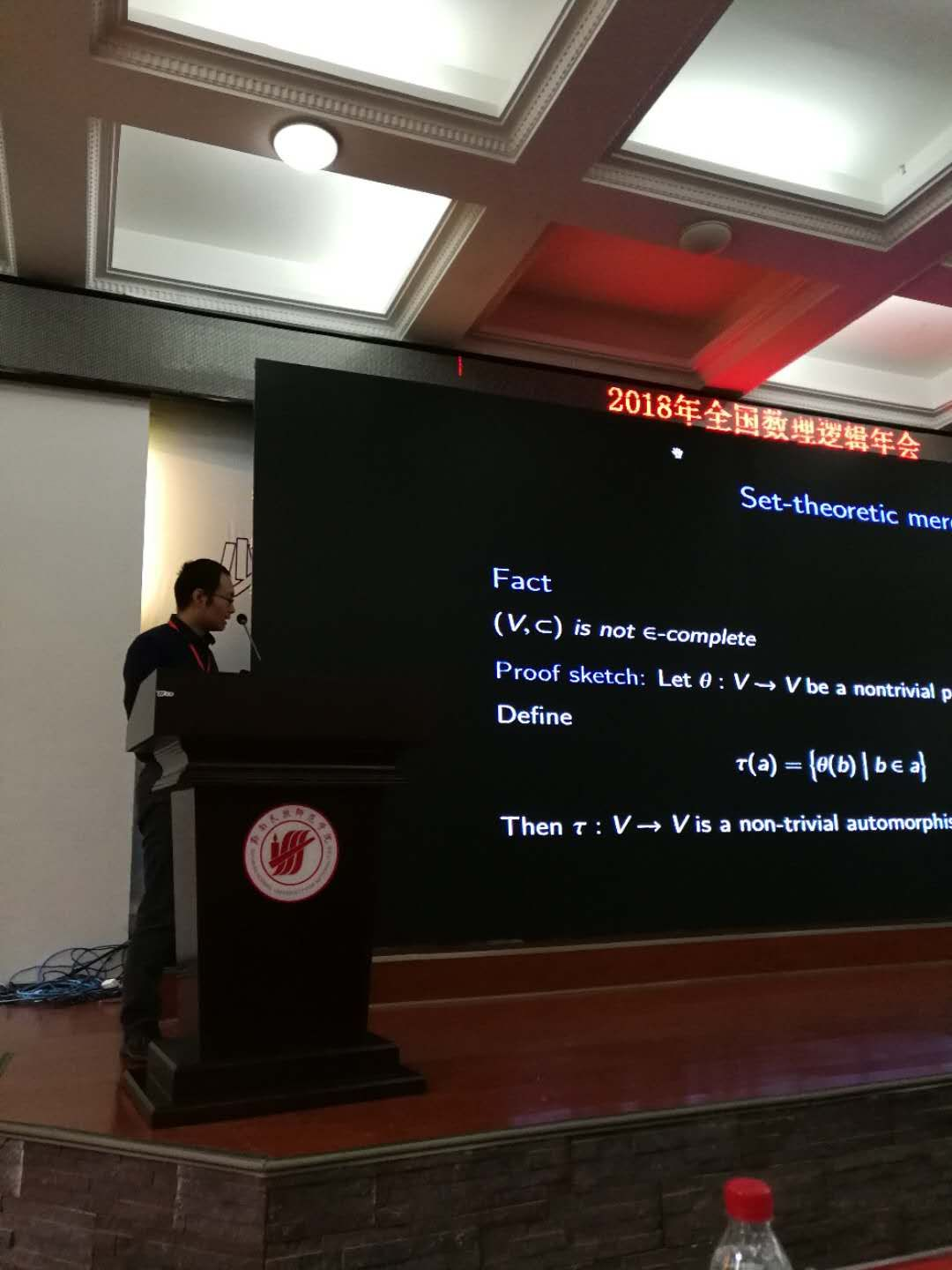## Chun’an, Zhejiang

Photographed by Wang Qiu. Scanned from films.

## How to define membership relation from subset relation and power set operationIn my talk at 2018 Chinese Mathematical Logic Conference, I asked if $$(V,\subset,P)$$ is epsilon-complete, namely if the membership relation can be recovered in the reduct. Professor Joseph S. Miller approached to me during the dinner and pointed out that it is epsilon-complete. Let me explain how.

Theorem

Let $$(V,\in)$$ be a structure of set theory, $$(V,\subset,P)$$ is the structure of the inclusion relation and the power set operation, which are defined in $$(V,\in)$$ as usual. Then $$\in$$ is definable in $$(V,\subset,P)$$.

Proof.

Fix a set $$x$$. Define $$y$$ to be the $$\subset$$-least such that

$\forall z \big((z\subset x\wedge z\neq x)\rightarrow P(z)\subset y\big).$

Actually, $$y=P(x)-\{x\}$$, so $$\{x\}= P(x) – y$$. Since set difference can be defined from subset relation and $$(V,\subset,\{x\})$$ can define $$\in$$, we are done.

$$\Box$$

Here is another argument figured out by Jialiang He and me after we heard Professor Miller’s Claim.

Proof.
Since $$\in$$ can be defined in $$(V,\subset,\bigcup)$$ (see the slides). Fix a set $$A$$, it suffices to show that we can define $$\bigcup A$$ from $$\subset$$ and $$P$$.

Let $$B$$ be the $$\subset$$-least set such that there is $$c$$, $$B=P(c)$$ and $$A\subset B$$. Note that
$\bigcap\big\{P(d)\bigm|A\subset P(d)\big\}= P\big(\bigcap\big\{d\bigm|A\subset P(d)\big\}\big).$
Therefore, $$B$$ is well-defined. Next, we show that
$\bigcap\big\{d\bigm|A\subset P(d)\big\}=\bigcup A.$
Clearly, $$A\subset P(\bigcup A)$$. This proves the direction from left to right. For the other direction, if $$x$$ is in an element of $$A$$, then it is in an element of $$P(d)$$ given $$A\subset P(d)$$, i.e. it is an element of such $$d$$.

Therefore $$\bigcup A$$ is the unique set whose power set is $$B$$.

$$\Box$$